Writing Recursive Subroutines

We note immediately that the IBM 370 does not directly support recursion.

The purpose of this lecture is to use the stack handling macros discussed
in a previous lecture to implement simple recursive subroutines.

Recursion is a process that requires a stack, provided either explicitly
or by the RTS (Run Time System).

Without native support for recursion, we must directly manage the call stack.

The simple protocol has two steps.

Subroutine Call:              Push the return address onto the stack

Branch to the subroutine

Subroutine Return           Pop the return address into a register

Other protocols provide for using the stack to transmit variables.
We shall discuss those later in this lecture.

NUMOUT: The Old Version

Here is the original code for the packed decimal version of NUMOUT.

NUMOUT CVD R4,PACKOUT           CONVERT TO PACKED

UNPK THENUM,PACKOUT      PRODUCE FORMATTED NUMBER

MVZ THENUM+7(1),=X’F0’   CONVERT SIGN HALF-BYTE

BR 8                     RETURN ADDRESS IN R8

*

This is the calling sequence for NUMOUT, placed within its context.

MVC PRINT,BLANKS      CLEAR THE OUTPUT BUFFER

BAL 8,NUMOUT          PRODUCE THE FORMATTED SUM

MVC DATAPR,THENUM     AND COPY TO THE PRINT AREA

Note that the BAL instruction saves the address of the next instruction into R8
before the branch is taken.

The saved return address is then used by the BR 8 instruction to return from the
subroutine.

NUMOUT: A Modest Revision

The newer version of NUMOUT will start the change to a style that is required
for recursive programming.

This style requires explicit management of the return address.  This requires
the definition of a label for the instruction following NUMOUT.

MVC PRINT,BLANKS      CLEAR THE OUTPUT BUFFER

LA  8,NUMRET          STATEMENT AFTER NUMOUT

B   NUMOUT            BRANCH DIRECTLY TO NUMOUT

NUMRET   MVC DATAPR,THENUM     AND COPY TO THE PRINT AREA

At this point, the NUMOUT code is not changed.

NUMOUT CVD R4,PACKOUT           CONVERT TO PACKED

UNPK THENUM,PACKOUT      PRODUCE FORMATTED NUMBER

MVZ THENUM+7(1),=X’F0’   CONVERT SIGN HALF-BYTE

BR 8                     RETURN ADDRESS IN R8

The newer version of NUMOUT will be written in the style required for recursive
subroutines, although it will not be recursive.

This style requires explicit management of the return address.  This requires
the definition of a label for the instruction following NUMOUT.

MVC PRINT,BLANKS      CLEAR THE OUTPUT BUFFER

LA  8,NUMRET          STATEMENT AFTER NUMOUT

STKPUSH R8,R          PLACE ADDRESS ONTO STACK

B   NUMOUT            BRANCH DIRECTLY TO NUMOUT

NUMRET   MVC DATAPR,THENUM     AND COPY TO THE PRINT AREA

Here is the new code for NUMOUT.

NUMOUT CVD R4,PACKOUT           CONVERT TO PACKED

UNPK THENUM,PACKOUT      PRODUCE FORMATTED NUMBER

MVZ THENUM+7(1),=X’F0’   CONVERT SIGN HALF-BYTE

STKPOP R8,R              POP THE RETURN ADDRESS

BR 8                     RETURN ADDRESS IN R8

Factorial: A Recursive Function

One of the standard examples of recursion is the factorial function.

We shall give its standard recursive definition and then show some typical code.

Definition:      If N £ 1, then  N! = 1

Otherwise        N! = N·(N – 1)!

Here is a typical programming language definition of the factorial function.

Integer Function FACT(N : Integer)

If N £ 1 Then Return 1

Else Return N*FACT(N – 1)

Such a function is easily implemented in a compiled high–level language (such as C++
or Java) that provides a RTS (Run Time System) with native support of a stack.

As we shall see, a low–level language, such as IBM 370 assembler, must be provided
with explicit stack handling routines if recursion is to be implemented.

Tail Recursion and Clever Compilers

Compilers for high–level languages can generally process a construct that is
“tail recursive”, in which the recursive call is the last executable statement.

Consider the above code for the factorial function.

Integer Function FACT(N : Integer)

If N £ 1 Then Return 1

Else Return N*FACT(N – 1)

Note that the recursive call is the last statement executed when N > 1.

A good compiler will turn the code into the following, which is equivalent.

Integer Function FACT(N : Integer)

Integer F = 1  ; Declare a variable and initialize it

For (K = 2, K++, K <= N) Do F = F*K ;

Return F ;

This iterative code consumes fewer RTS resources and executes much faster.

NOTE: For fullword (32–bit integer) arithmetic, the biggest we can calculate is 12!

A Simple Mechanism for Return
(How CDC–6400 FORTRAN did it)

We have already stated that management of the return address for a subroutine or
function will involve a stack.  We now investigate why this is the case.

The simplest method for storing the return address is to store it in the subroutine itself.

This mechanism allocates the first word of the subroutine to store the return address.

For a computer, such as the IBM/System 370, in which addresses are stored as
four–byte longwords, the structure would be as follows.

Address (Z + 4)       holds the first executable instruction of the subroutine.

BR *Z                     An indirect jump on Z is the last instruction of the subroutine.
Since Z holds the return address, this affects the return.

This is a very efficient mechanism.

The difficulty is that it cannot support recursion.

Example: Non–Recursive Call

Suppose the following instructions

100          JSR 200

101          Next Instruction

201          First Instruction

Last         BR *200

After the subroutine call, we would have

100          JSR 200

101          Next Instruction

200          101

201          First Instruction

Last         BR *200

The BR*200 would cause a branch to address 101, thus causing a proper return.

Example 2: Using This Mechanism Recursively

Suppose a five instruction subroutine at address 200.

This subroutine contains a single recursive call to itself that will be executed once.

Called from             First Recursive                First

200  101                  200  204                          200  204

201  Inst 1               201  Inst 1                       201  Inst 1

202  Inst 2               202  Inst 2                       202  Inst 2

203  JSR 200           203  JSR 200                   203  JSR 200

204  Inst 4               204  Inst 4                       204  Inst 4

205  BR * 200         205  BR * 200                 205  BR * 200

Note that the original return address has been overwritten.

As long as the subroutine is returning to itself, there is no difficulty.

Use a Stack to Hold Return Addresses

With the code above, we assume that a stack holds the return addresses.

Main calls the subroutine                SP ® 101

The subroutine calls itself               SP ® 204 ® 101

First return                                      SP ® 101

The subroutine returns to itself.

Second return

The subroutine returns to the main program.

Our design will use the following convention.

JSR          will push the return address to the stack.

RET         will pop the return address from the stack.

Implementation of the Stack Operations

Arbitrarily, I have decided that the stack grows toward more positive addresses.

Given this we have two options for implementing PUSH,
each giving rise to a unique implementation of POP.

Option         PUSH X                                  POP Y

1             M[SP] = X                              SP = SP – 1     // Post–increment on PUSH

SP = SP + 1                             Y = M[SP]

2             SP = SP + 1                             Y = M[SP]      // Pre–increment on PUSH

M[SP] = X                              SP = SP – 1

For a few reasons not related to this course, I have elected to implement the first option.

Post–increment on PUSH must be paired with pre–decrement on POP.

This is the option that was seen in my previous lecture on the stack macros
STKPUSH and STKPOP.

Outline of the Solution

Given the limitations of the IBM 370 original assembly language, the only way to
implement recursion is to manage the return addresses ourselves.

This must be done by explicit use of the stack.

Given that we are handling the return addresses directly,
we dispense with the BAL instruction and
use the unconditional branch instruction, B.

Here is code that shows the use of the unconditional branch instruction.
At this point, register R4 contains the argument.

LA R8,A94PUTIT  ADDRESS OF STATEMENT AFTER CALL

STKPUSH R8,R    PUSH THE ADDRESS ONTO THE STACK

STKPUSH R4,R    PUSH THE ARGUMENT ONTO THE STACK

B   DOFACT      CALL THE SUBROUTINE

A94PUTIT  BAL 8,NUMOUT    FINALLY, RETURN HERE.

Note that the address of the return instruction is placed on the stack.

Note also that the return target uses the traditional subroutine call mechanism.
We are assuming the original form of NUMOUT.

Proof of Principle: Code Fragment 1

Here is the complete code for the first proof of principle.

The calling code is as follows.  The function is now called DOFACT.

LA R8,A94PUTIT  ADDRESS OF STATEMENT AFTER CALL

STKPUSH R8,R    PUSH THE ADDRESS ONTO THE STACK

STKPUSH R4,R    PUSH THE ARGUMENT ONTO THE STACK

B   DOFACT      CALL THE SUBROUTINE

A94PUTIT  BAL 8,NUMOUT    FINALLY, RETURN HERE.

The code for this “do nothing” version of DOFACT is as follows.

DOFACT    STKPOP R4,R     POP ARGUMENT BACK INTO R4

STKPOP R8,R     POP RETURN ADDRESS INTO R8

BR 8            BRANCH TO THE POPPED ADDRESS

Remember:    1.     STKPOP R4,R is a macro invocation.

2.     The macros have to be written with a symbolic parameter as
the label of the first statement.

The Stack for Both Argument and Return Address

We now examine a slightly non–standard approach to using the stack to store both
arguments to the function and the return address.

In general, the stack can be used to store any number of arguments to a function or
subroutine.  We need only one argument, so that is all that we shall stack.

Remember that a stack is a     Last In / First Out data structure.

It could also be called a          First In / Last Out data structure; this is seldom done.

Recall the basic structure of the function DOFACT

DOFACT

Use the argument from the stack

STKPOP R8,R     POP RETURN ADDRESS INTO R8

BR 8            BRANCH TO THE POPPED ADDRESS

Since the return address is the last thing popped from the stack when the routine returns,
it must be the first thing pushed onto the stack when the routine is being called.

Basic Structure of the Function

On entry, the stack has both the argument and return address.

On exit, it must have neither.  The return address is popped last,
so it is pushed first.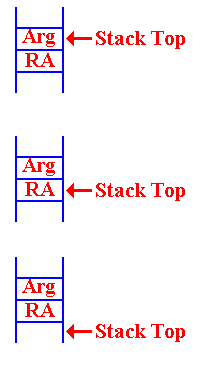On entry to the routine, this is the status of the stack.

By “Stack Top”, I indicate the location of the last item pushed.

At some point, the argument must be popped from the stack,

so that the Return Address is available to be popped.

STKPOP  8

BR  8

Note that the contents of the stack are not removed.

When Do We Pop the Argument?

The position of the STKPOP depends on the use of the argument sent to the function.

There are two considerations, both of which are quite valid.

Assume that register R7 contains the argument.  We shall get it there on the next slide.

Consider the fragment of code corresponding to N·FACT(N – 1).

FDOIT    S R7,=F'1'          SUBTRACT 1 FOR NEW ARGUMENT

LA  8,FRET          GET THE ADDRESS OF RETURN

STKPUSH  R8,R       STORE NEW RETURN ADDRESS

STKPUSH  R7,R       NOW, PUSH NEW ARG ONTO STACK

B DOFACT            MAKE RECURSIVE CALL

FRET     L R2,=F'0'

At this point, the return register (say R4) will contain FACT(N – 1).

At this point, the value of N should be popped from the stack and multiplied by the result
to get the result N
·FACT(N – 1), which will be placed into R4 as the return value.

When Do We Pop the Argument? (Part 2)

But recall that the basic structure of the factorial function calls for something like:

STKPOP R7,R

If the value in R7 is not greater than 1, execute this code.

L  R4,=F’1’   SET THE RETURN VALUE TO 1

STKPOP R8,R   POP THE RETURN ADDRESS

If the value in R7 is larger than 1, then execute this code.

FDOIT    S R7,=F'1'          SUBTRACT 1 FOR NEW ARGUMENT

LA  8,FRET          GET THE ADDRESS OF RETURN

STKPUSH  R8,R       STORE NEW RETURN ADDRESS

STKPUSH  R7,R       NOW, PUSH NEW ARG ONTO STACK

B DOFACT            MAKE RECURSIVE CALL

FRET     L R2,=F'0'

But, there is only one copy of the argument value.  How can we pop it twice.

Answer: We push it back onto the stack.

Examining the Value at the Top of the Stack

Here is the startup code for the function DOFACT.

DOFACT   STKPOP   R7,R       GET THE ARGUMENT AND EXAMINE

STKPUSH  R7,R       BUT PUT IT BACK ONTO THE STACK

C R7,=F'1'          IS THE ARGUMENT BIGGER THAN 1

BH  FDOIT           YES, WE HAVE A COMPUTATION

This code fragment shows the strategy for examining the top of the stack
without removing the value: just pop it and push it back onto the stack.

There is another common way of examining the top of the stack.

Many stack implementations use a function STKTOP, which returns the value at
the stack top without removing it.

This is another valid option.  That code could be written as follows.  Note that it uses
another stack operation, STKTOP, that I have not defined or used.

DOFACT   STKTOP  R7,R        GET THE ARGUMENT VALUE

C R7,=F'1'          IS THE ARGUMENT BIGGER THAN 1

BH  FDOIT           YES, WE HAVE A COMPUTATION

The Factorial Function DOFACT

DOFACT   STKPOP   R7,R       GET THE ARGUMENT AND EXAMINE

STKPUSH  R7,R       BUT PUT IT BACK ONTO THE STACK

C R7,=F'1'          IS THE ARGUMENT BIGGER THAN 1

BH  FDOIT           YES, WE HAVE A COMPUTATION

L R4,=F'1'          NO, JUST RETURN THE VALUE 1

STKPOP   R7,R       ARG IS NOT USED, SO POP IT

B FDONE             AND RETURN

FDOIT    S R7,=F'1'          SUBTRACT 1 FOR NEW ARGUMENT

LA  8,FRET          GET THE ADDRESS OF RETURN

STKPUSH  R8,R       STORE NEW RETURN ADDRESS

STKPUSH  R7,R       NOW, PUSH NEW ARG ONTO STACK

B DOFACT            MAKE RECURSIVE CALL

FRET     STKPOP   R7,R       POP THIS ARGUMENT FROM STACK

MR 6,4              PUT R4*R7 INTO (R6,R7)

LR 4,7              COPY PRODUCT INTO R4

*

*        THE FUNCTION VALUE IS ALWAYS RETURNED IN R4.

*

FDONE    STKPOP   R8,R       POP  RETURN ADDRESS FROM STACK

BR 8                BRANCH TO THAT ADDRESS

Analysis of DOFACT (Part 1)

At this point, the register R4 contains the return value of the function,
and the argument has been removed from the stack.

FDONE    STKPOP  R8,R    POP RETURN ADDRESS FROM STACK

BR 8            BRANCH TO THAT ADDRESS

The label FDONE is the common target address for the two cases discussed above.
Again, here is the top–level structure.

1.     Get the argument value, N, from the stack.

2.     If ( N £ 1 ) then
Set the return value to 1
B  FDONE

3.     If ( N ³ 2) then
Handle the recursive call and return from that call.

4.     FDONE: Manage the return from the function

DOFACT Part 2: Handling the Case for N £ 1

Here is the startup code and the code to return the value for N £ 1.

DOFACT   STKPOP   R7,R   GET THE ARGUMENT AND EXAMINE

STKPUSH  R7,R   BUT PUT IT BACK ONTO THE STACK

C R7,=F'1'      IS THE ARGUMENT BIGGER THAN 1

BH  FDOIT       YES, WE HAVE A COMPUTATION

*

* N = 1

L R4,=F’1’      NO, JUST RETURN THE VALUE 1

STKPOP  R7,R    ARG IS NOT USED, SO POP IT

B FDONE         AND RETURN

The startup code uses STKPOP followed by STKPUSH to get the argument
value into register R7 without removing it from the stack.

That value is then compared to the constant 1.

If the argument has value 1 or less, the return value is set at 1.
I arbitrarily define (–2)! to be 1.

Note that the argument is still on the stack.  It must be popped so that the return
address will be at the top of the stack and useable by the return code at FDONE.

DOFACT Part 3: Handling the Case for N > 1

Here is the code for the case N > 1.

FDOIT    S R7,=F'1'     SUBTRACT 1 FOR NEW ARGUMENT

LA  8,FRET     GET THE ADDRESS OF RETURN

STKPUSH  R8,R  STORE NEW RETURN ADDRESS

STKPUSH  R7,R  NOW, PUSH NEW ARG ONTO STACK

B DOFACT       MAKE RECURSIVE CALL

FRET     L R2,=F'0'     NON-MACRO STATEMENT AS TARGET

STKPOP R7,R    POP THIS ARGUMENT FROM STACK

*HERE

*     R7 CONTAINS THE VALUE N

*     R4 CONTAINS THE VALUE FACT(N – 1)

*

MR 6,4         PUT R4*R7 INTO (R6,R7)

LR 4,7         COPY PRODUCT INTO R4

The code then falls through to the “finish up” code at FDONE.

Note the structure of multiplication.  Remember that an even–odd register pair,
here (6, 7) is multiplied by another register.

Sample Run for DOFACT

We shall now monitor the state of the stack for a typical call to the recursive
function DOFACT.

Here is the basic structure for the problem.  First we sketch the calling code.

LA  8,A1       STATEMENT AFTER CALL TO SUBROUTINE

STKPUSH R8,R   PLACE RETURN ADDRESS ONTO STACK

B   DOFACT     BRANCH DIRECTLY TO SUBROUTINE

A1   The next instruction.

Here is the structure of the recursive function DOFACT

DOFACT   Check value of argument

Branch to FDONE if the argument < 2.

Call DOFACT recursively

FRET     Return address for the recursive call

FDONE    Close–up code for the subroutine

More on the Stack

We now relate the idea of the Stack Top to our use of the SP (Stack Pointer).

The protocol used for stack management is called “post increment on push”.

In a high level programming language, this might be considered as follows.

PUSH ARG   M[SP] = ARG         POP ARG   SP = SP – 1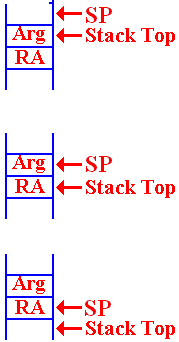SP = SP + 1                   ARG = M[SP]

The status of the stack is always that the SP points to the location
into which the next item pushed will be placed.

On entry to the function, there is an argument on the top of the
stack.  The return address is the value just below the argument.

When the argument is popped from the stack, we are left with the SP
pointing to the argument value that has just been popped.  The return
address (RA) is now on the stack top and available to be popped.

After the RA has been popped, the SP points to its value.
Whatever had been on the stack is now at the Stack Top.

Consider DOFACT For the Factorial of 3

Remember our notation for return addresses:    A1 for the calling routine.

FR for the return in DOFACT.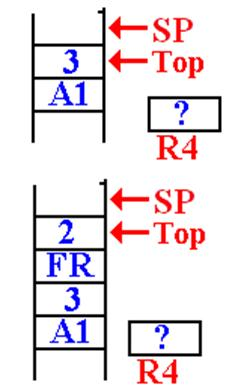This is the status of the stack when DOFACT is first called.

The return address (A1) of the main program was pushed
first, and then the value (3) was pushed.

The value in R4, used for the return value, is not important.

It is noted that 3 > 1 so DOFACT will be called with a value
of 2.  When the first recursive call is made, the stack status is
shown at left.

The top of the stack has the value 2.
The return address (FR) of the DOFACT function was
first pushed, followed by the argument value.

The Next Recursive Call To DOFACT

On the next call to DOFACT, the value at the top of the stack is found to be 2.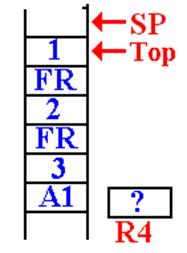It is noted that 2 > 1.

The argument value for the next recursive call is computed,

The return address (FR) for DOFACT is pushed onto the stack.
Then the value of the new argument (1) is pushed onto the stack.

DOFACT is called again.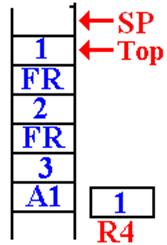In this next call to DOFACT, the value at the top of the stack is
examined and found to be 1.

A return value is placed into the register R4, which has been
reserved for that use.

This is the status of the stack just before the first return.

The First Recursive Return

The first recursive return is to address FR (or FRET) in DOFACT.

Here is the situation just after the first recursive return.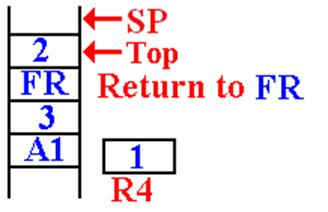The argument value for this invocation is
now at the top of the stack.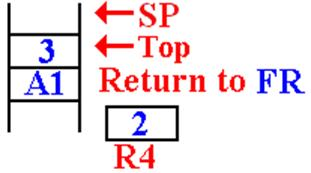The value 2 is removed from the stack, multiplied
by the value in R4 (which is 1) and then stored in R4.

stack.  The function returns to itself.

The Next Recursive Return

The next recursive return is to address FR (or FRET) in DOFACT.

Here is the situation just after the first recursive return.Here is the status of the stack after this
return.  The argument value is on the top
of the stack, followed by the return address
for the main routine.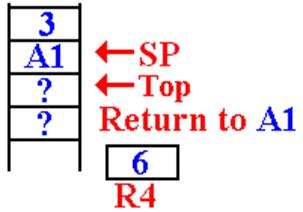On the final return, the value 3 has been removed
from the stack, multiplied by the value in R4, and
the new function value (6) is placed back into R4.

The return address (A1) has been popped from the
stack and the function returns there.

When a subroutine or function is called, control passes to that subroutine but must return
to the instruction immediately following the call when the subroutine exits.

There are two main issues in the design of a calling mechanism for

1.     How to pass the return address to the subroutine so that, upon completion,
it returns to the correct address.  We have just discussed this problem.

2.     How to pass the arguments to the subroutine and return values from it.

A function is just a subroutine that returns a value.  For functions, we have one additional
issue in the linkage discussion: how to return the function value.

This presentation will be a bit historical in that it will pose a number of linkage
mechanisms in increasing order of complexity and flexibility.

We begin with a simple mechanism based on early CDC–6600 FORTRAN compilers.

Pass–By–Value and Pass–By–Reference

Modern high–level language compilers support a number of mechanisms for passing
arguments to subroutines and functions.  These can be mimicked by an assembler.

Two of the most common mechanisms are:

1.     Pass by value, and

2.     Pass by reference.

In the pass–by–value mechanism, the value of the argument is passed to the subroutine.

In the pass–by–reference, it is the address of the argument that is passed to the subroutine,
which can then modify the value and return the new value.

Suppose that we want to use register R10 to pass an argument to a subroutine.
That argument is declared as follows.

FW1    DC F‘35’

The operative code would be as follows:

Pass by value:             L  R10,FW1   Load the value at FW1

Returning Function Values

There is a simple solution here that is motivated by two facts.

1.   The function stores its return value as its last step.

2.   The first thing the calling code should do is to retrieve that value.

This simple solution is to allocate one or more registers to return function values.

There seem to be no drawbacks to this mechanism.  As we have seen above, it
works rather well with recursive functions.

The solution used in these lectures was to use R7 to return the value.

The CDC–6600 FORTRAN solution was to use one or two registers as needed.

Register R7     would return either a single–precision result or the
low–order bits of a double–precision result.

Register R6    would return the high–order bits of the double–precision result.

CDC Nerds note that the actual register names are X6 and X7.

Argument Passing: Version 1
(Based on Early CDC–6400 FORTRAN)

Pass the arguments in the general–purpose registers.  Here we use the
actual names of the registers: X0 through X7.

Register X0 was not used for a reason that I cannot remember.

Registers X1 through X5 are used to pass five arguments.

Registers X6 and X7 are used to return the value of a function.

This is a very efficient mechanism for passing arguments.

The problem arises when one wants more than five arguments to be passed.

There is also a severe problem in adapting this scheme to recursive subroutines.
We shall not discuss this at present for two reasons.

1.   We shall meet the identical problem later, in a more general context.

2.   None of the CDC machines was designed to support recursion.

Argument Passing: Version 2
(Based on Later CDC–6400 FORTRAN)

In this design, only two values are placed on the stack.  Each is an address.

The address of a memory block containing the number of arguments
and an entry (value or address) for each of the arguments.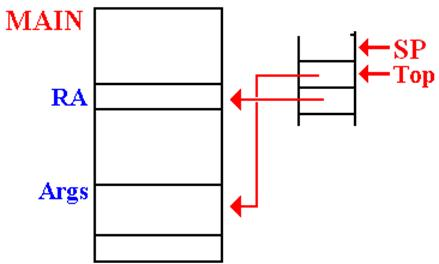This method allows for passing a large number of arguments.

This method can be generalized to be compatible with the modern stack–based protocols.

Example Code Based on CDC–6600 FORTRAN

Here is IBM/System 370 assembly language written in the form
that the CDC FORTRAN compiler might have emitted.

Consider a function with three arguments.  The call in assembly language might be.

LA  R8,FRET         ADDRESS OF STATEMENT TO BE
EXECUTED NEXT.

STKPUSH R8,R        PLACE ADDRESS ONTO STACK

STKPUSH R8,R        PLACE THAT ONTO THE STACK

B   THEFUNC         BRANCH DIRECTLY TO SUBROUTINE

A0       DC F‘3’             THE NUMBER OF ARGUMENTS

A1       DS F                HOLDS THE FIRST ARGUMENT

A2       DS F                HOLDS THE SECOND ARGUMENT

A3       DS F                HOLDS THE THIRD ARGUMENT

FRET     The instruction to be executed on return.

This cannot be used with recursive subroutines or functions.

The Solution: Use a Stack for Everything

We now turn our attention to a problem associated with writing a compiler.

The specifications for the high–level language state that recursion is to be supported,
both for subroutines and functions.

It is very desirable to have only one mechanism for subroutine linkage.

Some architectures, such as the VAX–11/780 did support multiple linkages,
but a compiler writer would not find that desirable.

We have a number of issues to consider:

1.   How to handle the return address.  This, we have discussed.

2.   How to handle the arguments passed to the subroutine or function.

We have just mentioned this one.

3.   How to handle the arguments and values local to the subroutine or function.

As we shall see next time, the answer is simple: Put everything on the stack.